Next: MINIMUM PHASE Up: One-sided functions Previous: One-sided functions

# INVERSE FILTERS

To understand causal filters better, we now take up the task of undoing what a causal filter has done. Consider the output yt of a filter bt is known but the input xt is unknown. See Figure 1.

 2-1 Figure 1 Sometimes the input to a filter is unknown.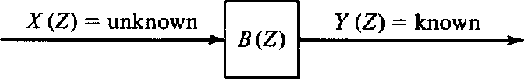This is the problem that one always has with a transducer/recorder system. For example, the output of a seismometer is a wiggly line on a piece of paper from which the seismologist may wish to determine the displacement, velocity, or acceleration of the ground. To undo the filtering operation of the filter B(Z), we will try to find another filter A(Z) as indicated in Figure 2.

 2-2 Figure 2 The filter A(Z) is inverse to the filter B(Z).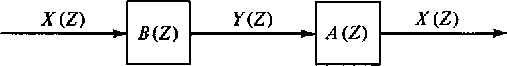To solve for the coefficients of the filter A(Z), we merely identify coefficients of powers of Z in B(Z)A(Z) = 1. For B(Z), a three-term filter, this is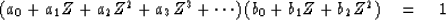(1)
The coefficients of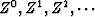in (1) are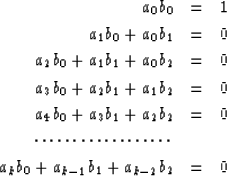(2) (3) (4) (5) (6) (7)
From (2) one may get a0 from b0. From (3) one may get a1 from a0 and the bk. From (4) one may get a2 from a1, a0, and the bk. Likewise, in the general case ak may be found from ak-1, ak-2, and the bk. Specifically, from (7) the ak may be determined recursively by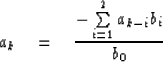(8)

Consider the example where B(Z) = 1 - Z/2; then, by equations like (2) to (7), by the binomial theorem, by polynomial division, or by Taylor's power series formula we obtain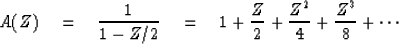(9)
We see that there are an infinite number of filter coefficients but that they drop off rapidly in size so that approximation in a computer presents no problem. The situation is not so rosy with the filter B(Z) = 1 - 2Z. Here we obtain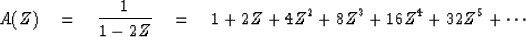(10)
The coefficients of the series increase without bound. The outputs of the filter A(Z) depend infinitely strongly on inputs of the infinitely distant past. [Recall that the present output of A(Z) is a0 times the present input x1 plus a1 times the previous input xt-1, etc., so an represents memory on n time units earlier.] The implication of this is that some filters B(Z) will not have useful finite approximate inverses A(Z) determined from (2) to (8). We now seek ways to identify the good filters from the bad ones. With a two-pulse filter, the criterion is merely that the first pulse in B(Z) be larger than the second. A more mathematical description of the state of affairs results from solving for the roots of B(Z), that is, find the values of Z0 for which B(Z0) = 0. For example 1 - Z/2 we find Z0 = 2. For the example 1 - 2Z, we find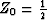.The general case for wavelets with complex coefficients is that, if the solution value Z0 of B(Z0) = 0 lies inside the unit circle in the complex plane, then 1/B(Z) will have coefficients which blow up; and if the root lies outside the unit circle, then the inverse 1/B(Z) will be bounded.2-3
Figure 3
Factoring the polynomial B(Z) breaks the filter into many two-term filters. Each one should have a bounded inverse.

Recalling earlier discussion that a polynomial B(Z) of degree N may be factored into N subsystems and that the ordering of subsystems is unimportant (see Figure 3), we suspect that if any of the N roots of B(Z) lies inside the unit circle we may have difficulty with A(Z). Actual proof of this suspicion relies on a theorem from complex-variable theory about absolutely convergent series. The theorem is that the product of absolutely convergent series is convergent, and conversely the product of any convergent series with a divergent series is divergent. Another proof may be based upon the fact that a power series for 1/B(Z) converges in a circle about the origin with a radius from the origin out to he first pole [the zero of B(Z) of smallest magnitude]. Convergence of A(Z) on the unit circle means, in terms of filters, that the coefficients of A(Z) are decreasing. Thus, if all the zeros of B(Z) are outside the unit circle, we will get a convergent filter from (8).

Can anything at all be done if there is one root or more inside the circle? An answer is suggested by the example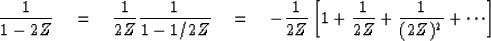(11)
Equation (11) is a series expansion in 1/Z, that is, a Taylor series about infinity. It converges from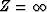all the way in to a circle of radius 1/2. This means that the inverse converges on the unit circle where it must, if the coefficients are to be bounded. In terms of filters it means that the inverse filter must be one of those filters which responds to future inputs and hence is not physically realizable but may be used in computer simulation.

In the general case, then, one must factor B(Z) into two parts: B(Z) = Bout(Z) Bin(Z) where Bout contains roots outside the unit circle and Bin contains the roots inside. Then the inverse of Bout is expressed as a Taylor series about the origin and the inverse of Bin is expressed as a Taylor series about infinity. The final expression for 1/B(Z) is called a Laurent expansion for 1/B(Z), and it converges on a ring surrounding the unit circle. Cases with zeros exactly on the unit circle present special problems. Sometimes you can argue yourself out of the difficulty but at other times roots on or even near the circle may mean that a certain computing scheme won't work out well in practice.

Finally, let us consider a mechanical interpretation. The stress (pressure) in a material may be represented by xt, and the strain (volume charge) may be represented by yt. The following two statements are equivalent; that is, in some situations they are both true, and in other situations they are both false:

STATEMENT A The stress in a material may be expressed as a linear combination of present and past strains. Likewise, the strain may be deduced from present and past stresses.

STATEMENT B The filter which relates stress to strain and vice versa has all poles and zeros outside the unit circle.

## EXERCISES:

1. Find the filter which is inverse to (2 - 5Z + 2Z2). You may just drop higher-order powers of Z, but an exact expression for the coefficients of any power of Z is preferred. (Partial fractions is a useful, though not necessary, technique.) Sketch the impulse response.
2. Show that multiplication by (1 - Z) in discretized time is analogous to time differentiation in continuous time. Show that dividing by (1 - Z) is analogous to integration. What are the limits on the integral?
3. Describe a general method for determining A(Z) and B(Z) from a Taylor series of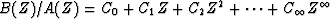where B(Z) and A(Z) are polynomials of unknown degree n and m, respectively. Work out the case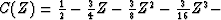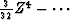. Don't try this problem unless you are quite familiar with determinants. [HINT: Identify coefficients of B(Z) = A(Z) C(Z).]Next: MINIMUM PHASE Up: One-sided functions Previous: One-sided functions
Stanford Exploration Project
10/30/1997